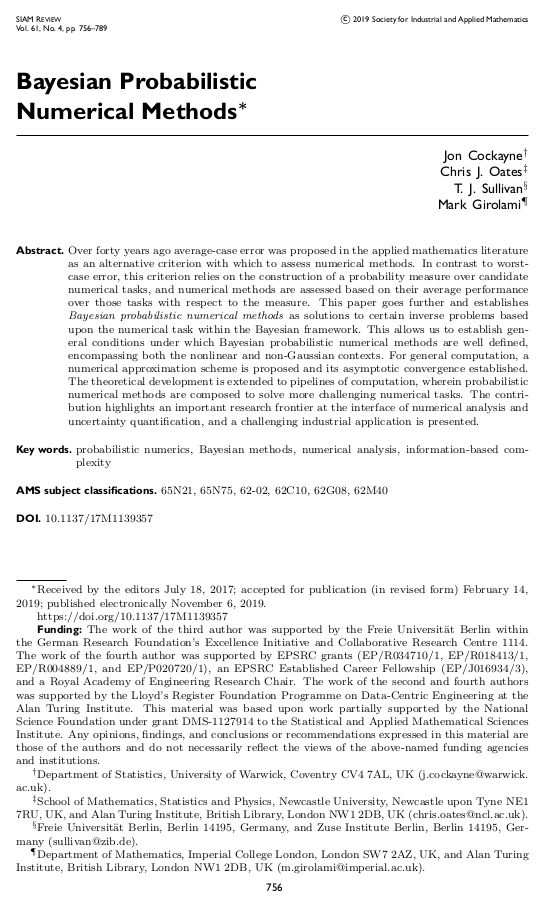# Tim Sullivan### Bayesian probabilistic numerical methods in SIAM Review

The 2019 Q4 issue of SIAM Review will carry an article by Jon Cockayne, Chris Oates, Mark Girolami, and myself on the Bayesian formulation of probabilistic numerical methods, i.e. the interpretation of deterministic numerical tasks such as quadrature and the solution of ordinary and partial differential equations as (Bayesian) statistical inference tasks.

J. Cockayne, C. J. Oates, T. J. Sullivan, and M. Girolami. “Bayesian probabilistic numerical methods.” SIAM Review 61(4):756–789, 2019. doi:10.1137/17M1139357

Abstract. Over forty years ago average-case error was proposed in the applied mathematics literature as an alternative criterion with which to assess numerical methods. In contrast to worst-case error, this criterion relies on the construction of a probability measure over candidate numerical tasks, and numerical methods are assessed based on their average performance over those tasks with respect to the measure. This paper goes further and establishes Bayesian probabilistic numerical methods as solutions to certain inverse problems based upon the numerical task within the Bayesian framework. This allows us to establish general conditions under which Bayesian probabilistic numerical methods are well defined, encompassing both the nonlinear and non-Gaussian contexts. For general computation, a numerical approximation scheme is proposed and its asymptotic convergence established. The theoretical development is extended to pipelines of computation, wherein probabilistic numerical methods are composed to solve more challenging numerical tasks. The contribution highlights an important research frontier at the interface of numerical analysis and uncertainty quantification, and a challenging industrial application is presented.

Published on Thursday 7 November 2019 at 07:00 UTC #publication #bayesian #siam-review #prob-num #cockayne #girolami #oates